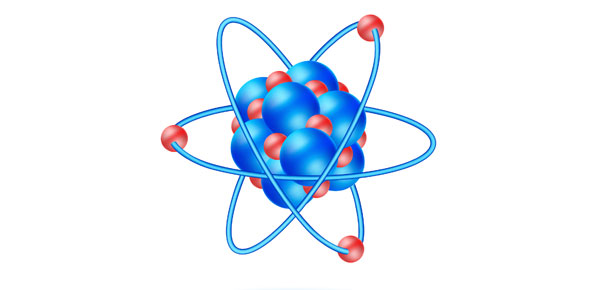# Physical Science - Part I

35 Questions | Total Attempts: 97SettingsPhysical Science6 weeks TestPart I

• 1.
Which of the following is NOT a physical property?
• A.

Sight

• B.

Color

• C.

Bases

• D.

Length

• 2.
Which of the following is the shortest measurement?
• A.

Millimeter

• B.

Centimeter

• C.

Meter

• D.

Kilometer

• 3.
_________ is a temporary magnet that can be turned on and off.
• A.

Electricitymagnet

• B.

Electionmagnet

• C.

Elevatedmagnet

• D.

Electromagnet

• 4.
What kind of energy do we get from the sun?
• A.

Icy

• B.

Factored

• C.

Polar

• D.

Solar

• 5.
Electricl energy can be changed into heat energy.
• A.

True

• B.

False

• 6.
Light travels the fastest through a ________.
• A.

Vacuum

• B.

Solid

• C.

Liquid

• D.

Gas

• 7.
Which of the following is NOT a conductor?
• A.

Steel

• B.

Wood

• C.

Copper

• D.

Iron

• 8.
___________ is the field around a magnet.  You can not see it, but you can see its effects.
• A.

Spark field

• B.

Baseball field

• C.

Attraction field

• D.

Magnetic field

• 9.
Which of the following is an example of a concave lens?
• A.

Glasses

• B.

Hand lens

• C.

Telescope

• D.

Microscope

• 10.
What happens when electrons move from one object to another object and stick onto the second object?
• A.

Static electricity

• B.

Moving electricity

• C.

• D.

Stationary electricity

• 11.
Which of the following describes a solid?
• A.

Packed loosely together

• B.

Flow and slide past each other

• C.

Packed tightly together in straight rows

• D.

Used in flourescent lights

• 12.
What would it take for matter to move from one state to another?
• A.

Energy

• B.

Matter

• C.

Help

• D.

This is impossible

• 13.
Which of the following is an insulator?
• A.

Iron

• B.

Plastic

• C.

Steel

• D.

Metal

• 14.
Sound is produced by ________________.
• A.

Staring

• B.

Talking

• C.

• D.

Vibrations

• 15.
Which measurement would you use to find the length of our classroom?
• A.

Kilometer

• B.

Meter

• C.

Centimeter

• D.

Millimeter

• 16.
How many volts are in the average wall outlet plug?
• A.

1.5 volts

• B.

150 volts

• C.

1.20 volts

• D.

120 volts

• 17.
Refraction means to ____________.
• A.

Bend light as it moves from one material to another

• B.

Bounce light from the ceiling to the floor

• C.

Cut the light with a laser beam

• D.

Find light in an unexpected place

• 18.
Draw a picture of light reflecting off of an object at an ANGLE.
• 19.
Name an object that is controlled by a switch.
• 20.
Draw a closed circuit.  You must label ALL of the parts and show the direction of the flow of electrons.
• 21.
What does the following picture show?
• A.

Small circuit

• B.

Half circuit

• C.

Short circuit

• D.

Burnt circuit

• 22.
Sunlight is _______ light.
• A.

Red

• B.

White

• C.

Blue

• D.

Violet

• 23.
Sound moves the fastest through a _______.
• A.

Liquid

• B.

Solid

• C.

Cup

• D.

Gas

• 24.
Which of the following would NOT be attracted to a magnet?
• A.

Another magnet

• B.

Rubber band

• C.

• D.

Paperclip

• 25.
Which of the following is a chemical change?
• A.

Clothes drying in the sun

• B.

Making chocolate milk

• C.

Shooting off fireworks

• D.Back to top